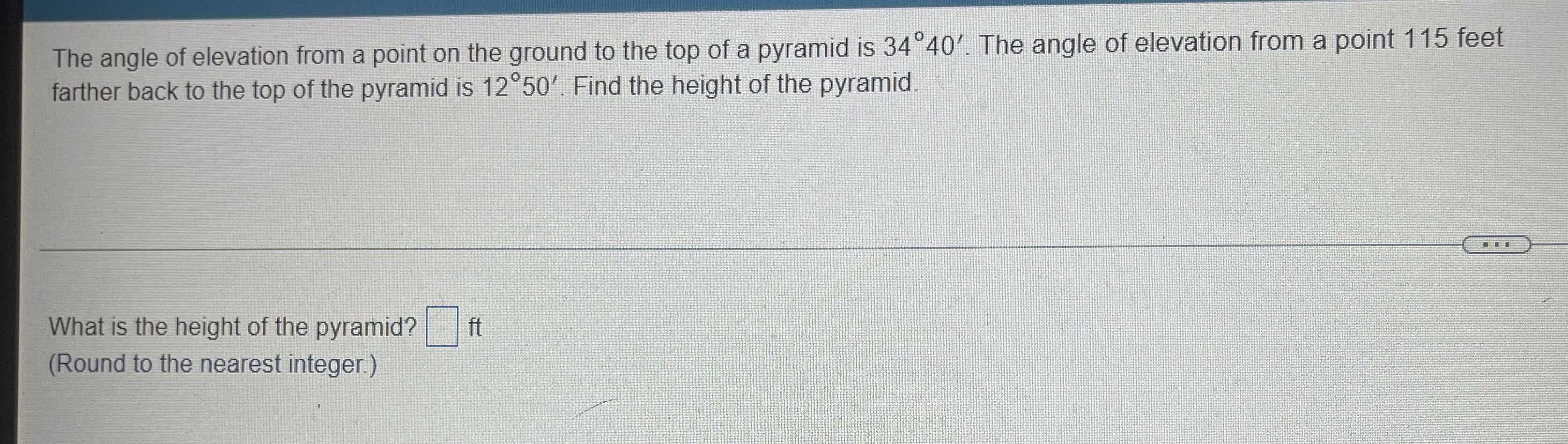### Still have math questions?

Trigonometry
QuestionThe angle of elevation from a point on the ground to the top of a pyramid is $$34 ^ { \circ } 40 ^ { \prime }$$ . The angle of elevation from a point $$115$$ feet farther back to the top of the pyramid is $$12 ^ { \circ } 50 ^ { \prime }$$ . Find the height of the pyramid.

What is the height of the pyramid? $$\square$$ ft

(Round to the nearest integer.)